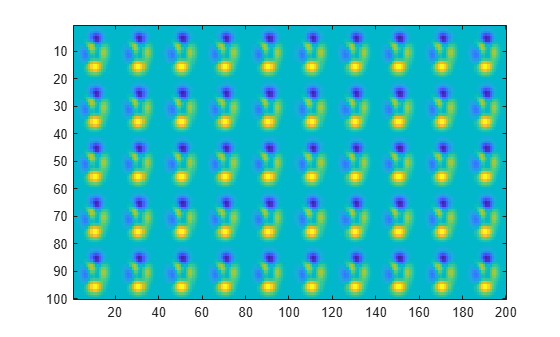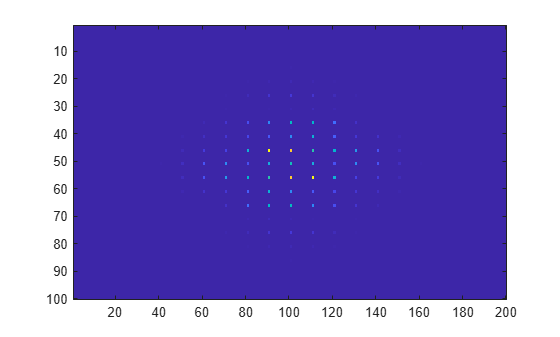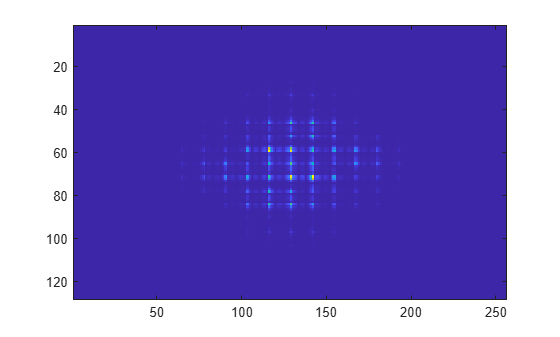fft2

2-D fast Fourier transform

Description

example

Y = fft2(X) returns the two-dimensional Fourier transform of a matrix using a fast Fourier transform algorithm, which is equivalent to computing fft(fft(X).').'. If X is a multidimensional array, then fft2 takes the 2-D transform of each dimension higher than 2. The output Y is the same size as X.

example

Y = fft2(X,m,n) truncates X or pads X with trailing zeros to form an m-by-n matrix before computing the transform. Y is m-by-n. If X is a multidimensional array, then fft2 shapes the first two dimensions of X according to m and n.

Examples

collapse all

The 2-D Fourier transform is useful for processing 2-D signals and other 2-D data such as images.

Create and plot 2-D data with repeated blocks.

P = peaks(20);
X = repmat(P,[5 10]);
imagesc(X)Compute the 2-D Fourier transform of the data. Shift the zero-frequency component to the center of the output, and plot the resulting 100-by-200 matrix, which is the same size as X.

Y = fft2(X);
imagesc(abs(fftshift(Y)))Pad X with zeros to compute a 128-by-256 transform.

Y = fft2(X,2^nextpow2(100),2^nextpow2(200));
imagesc(abs(fftshift(Y)));Input Arguments

collapse all

Input array, specified as a matrix or a multidimensional array. If X is of type single, then fft2 natively computes in single precision, and Y is also of type single. Otherwise, Y is returned as type double.

Data Types: double | single | int8 | int16 | int32 | uint8 | uint16 | uint32 | logical
Complex Number Support: Yes

Number of transform rows, specified as a positive integer scalar.

Data Types: double | single | int8 | int16 | int32 | uint8 | uint16 | uint32 | logical

Number of transform columns, specified as a positive integer scalar.

Data Types: double | single | int8 | int16 | int32 | uint8 | uint16 | uint32 | logical

collapse all

2-D Fourier Transform

This formula defines the discrete Fourier transform Y of an m-by-n matrix X:

${Y}_{p+1,q+1}=\sum _{j=0}^{m-1}\sum _{k=0}^{n-1}{\omega }_{m}^{jp}{\omega }_{n}^{kq}{X}_{j+1,k+1}$

ωm and ωn are complex roots of unity:

$\begin{array}{l}{\omega }_{m}={e}^{-2\pi i/m}\\ {\omega }_{n}={e}^{-2\pi i/n}\end{array}$

i is the imaginary unit. p and j are indices that run from 0 to m–1, and q and k are indices that run from 0 to n–1. This formula shifts the indices for X and Y by 1 to reflect matrix indices in MATLAB®.